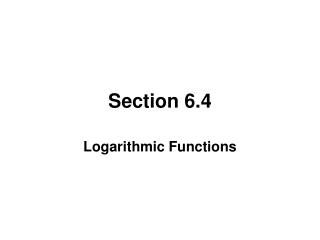DownloadDownload PresentationSection 6.4

# Section 6.4

Download Presentation## Section 6.4

- - - - - - - - - - - - - - - - - - - - - - - - - - - E N D - - - - - - - - - - - - - - - - - - - - - - - - - - -
##### Presentation Transcript

1. Section 6.4 Logarithmic Functions

2. LOGARITHIMS Since exponential functions are one-to-one, each has an inverse. These exponential functions are called logarithms.

3. LOGARITHMIC FUNCTIONS The logarithmic function to the base a, where a > 0 and a ≠ 0, is denoted by y = logax (read as “y is the logarithm to the base a of x”) and is defined by y = loga x if and only if x = a y The domain of the logarithmic function y =loga x is x > 0.

4. EXPONENTIAL AND LOGARITHMIC FORMS • The exponential form of y = logbx is by = x. • The logarithmic form of by = x is y = logbx.

5. GRAPHING LOGARITHMIC FUNCTIONS To quickly graph the logarithmic function y = logb x plot points for x = 1/b, 1, and b.

6. PROPERTIES OF f (x) = logb x • Domain: (0, ∞) • Range: (−∞, ∞) • The x-intercept of the graph is 1. There is no y-intercept. • Vertical Asymptote: x = 0 • Increasing if b > 1 • Decreasing if 0 < b < 1

7. DOMAIN OF A LOGARITHMIC FUNCTIONS Since the logarithm of a negative number and the logarithm of zero cannot be taken, the argument of a logarithmic function must always be positive. That is, if Z is an algebraic expression in x, the domain of f (x) = logb Z is the set of numbers such that Z > 0.

8. COMMON AND NATURAL LOGARITHMS Logarithms with a base of 10 are called common logarithms. We denote this by log x. That is, log x = log10x. Logarithms with a base of e are called natural logarithms. We denote this by ln x. That is, ln x = logex.

9. LOGARITHMIC EQUATIONS Equations that contain logarithms are called logarithmic equation. Some logarithmic equations can be solved by converting them to exponential form. However, when solving logarithmic equations, you must always check your solutions.